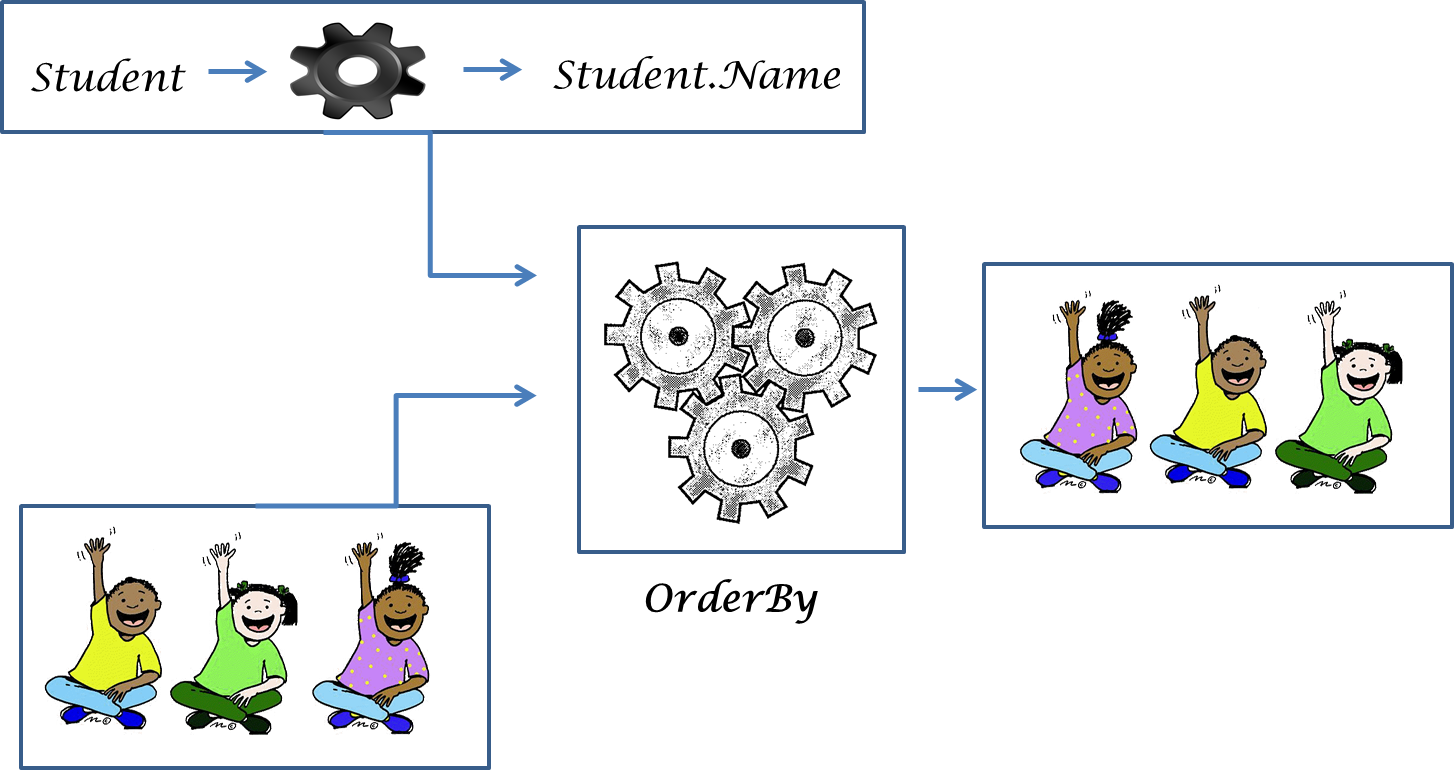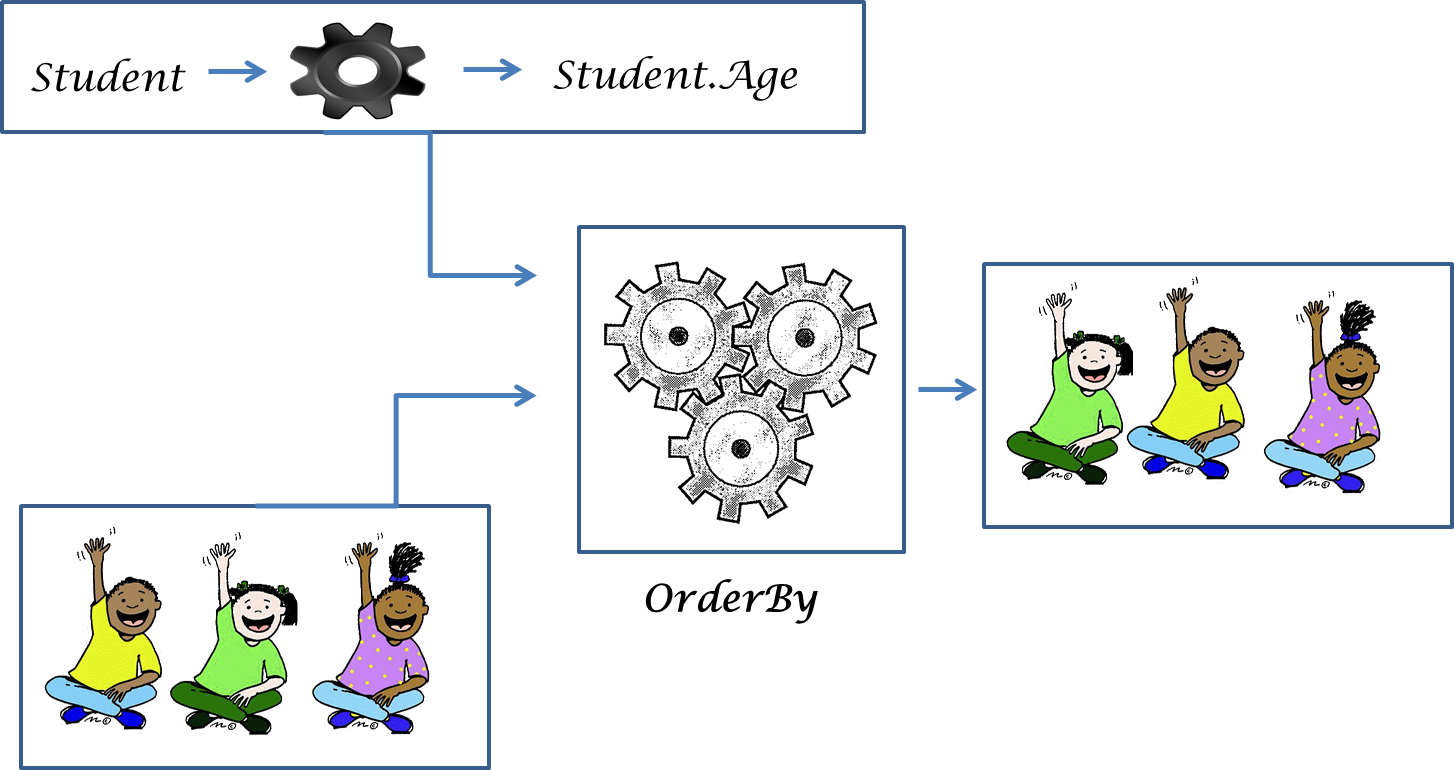# Verbs - Buried Abstraction in OO World

Published on

In Object Oriented Programming, the problem domain is being modeled as objects(nouns) which collaborate with one another in the form of messages(verbs). We are actually creating abstractions and through which we are solving the problems in an organized and cleaner way. But this way of creating abstractions does not scale in all the scenarios.

Let me share it through an example

Lets rewind the time machine to travel back to 2006 (.NET 2.0) which doesn’t have `LINQ` support. You are asked to solve the following problem statement

You are working on a Student Management Application. Each student is having a name and an age. You are asked to create module to sort the Students based on their name

``public class Student{  public int Age { get; set; }  public string Name { get; set; }}``

To sort based on Name we need to write a comparer which compares two student names and return some integer.

``public class StudentNameComparer : IComparer<Student>{  public int Compare(Student x, Student y)  {    return string.Compare(x.Name, y.Name, StringComparison.CurrentCulture);  }}``

Then in the `main` program we will be doing the sorting using Array.Sort method

``class Program{  static void Main(string[] args)  {    List<Student> students = new List<Student>();    // Populate Students List here	    Student[] studentsSortedByName = students.ToArray();    Array.Sort(studentsSortedByName, new StudentNameComparer());  }}``

Hmmm. You might think what is a big deal in it ? But there is! Let me complicate the scenario by adding a new requirement

You also asked to do sorting based on Age!

How do you do it ?

Well! Just create yet another Comparer which compares the age

``public class StudentAgeComparer : IComparer<Student>{  public int Compare(Student x, Student y)  {    if (x.Age == y.Age)    {      return 0;    }    if (x.Age < y.Age)    {      return -1;    }      return 1;  }}``

So much of code to do the trivial thing, isn’t it ?

Let us dig deep to figure out what is wrong here. First let us start with the comparers `StudentAgeComparer` and `StudentNameComparer`. These comparers (nouns) actually escorting the method `Compare` (verb) and not doing anything productive apart from this. In OO world a verb should always be associated with a noun and because of this way of abstraction, we are adding an unnecessary complexity to the code.

Just think of yet another scenario where want to sort the student by rank, customer by name, product by name. We need to write so much comparers!

## Verbs to the rescue

Let us see the student sorting scenario from a different perspective. In this new pair of (functional)eyes we see the same scenario as

• Order Student by Name
• Order Student by Age
• Order Customer by Name
• Order Product by Name

i.e the verb Order is common across all the scenario.

The only thing which is changing is what we are ordering and by which property. Let us model things based on this verb abstraction.

PseudoCode

``OrderBy<Student>(s -> s.Name)OrderBy<Student>(s -> s.Age)OrderBy<Customer>(c -> c.Name)OrderBy<Product>(p -> p.Name)``

Here `OrderBy` is a function which models the verb (Order). Two things to note

`OrderBy` is generic and it can act on any types of objects (`Student`, `Customer`, `Product`,…) `OrderBy` doesn’t know which property to choose so, we need to tell explicitly.

So, what we gained because of this ?

Let us go back to the initial requirement

You are working on a Student Management Application. Each student is having a name and an age. You are asked to create module to sort the Students based on their name and age

Now change the time machine to land in 2014. We have `LINQ` support in C# now which supports verbs (functions) as first class citizens

``class Program2{  static void Main(string[] args)  {    List<Student> students = new List<Student>();    // Populate Students List here	    var studentsSortedByName = Enumerable.OrderBy(students, s => s.Name);    var studentsSortedByAge = Enumerable.OrderBy(students, s => s.Age);  }}``

Just two lines instead of two comparers. All we did is just changed the way we create abstractions. Let me explain it using some visuals.`OrderBy` is an abstraction which does only one thing, that is order the objects in an ascending order and does it to the perfection. But to use this abstraction we need to provide two inputs, the objects and a function which provides the propery by which the objects to be sorted

Does it closely resemble what we have seen earlier in the pseudocode ? Since it is generic, we can also use it for all other objects (Product, Customer, etc.,) too !## Summary

Verbs(functions) are not silver bullets and they are not the replacement of Nouns(objects). But by choosing our abstraction wisely we can achieve great things with lesser lines of code and in turn release the code with lesser bugs 😄

Did the content capture your interest? Stay in the loop by subscribing to the RSS feed and staying informed!